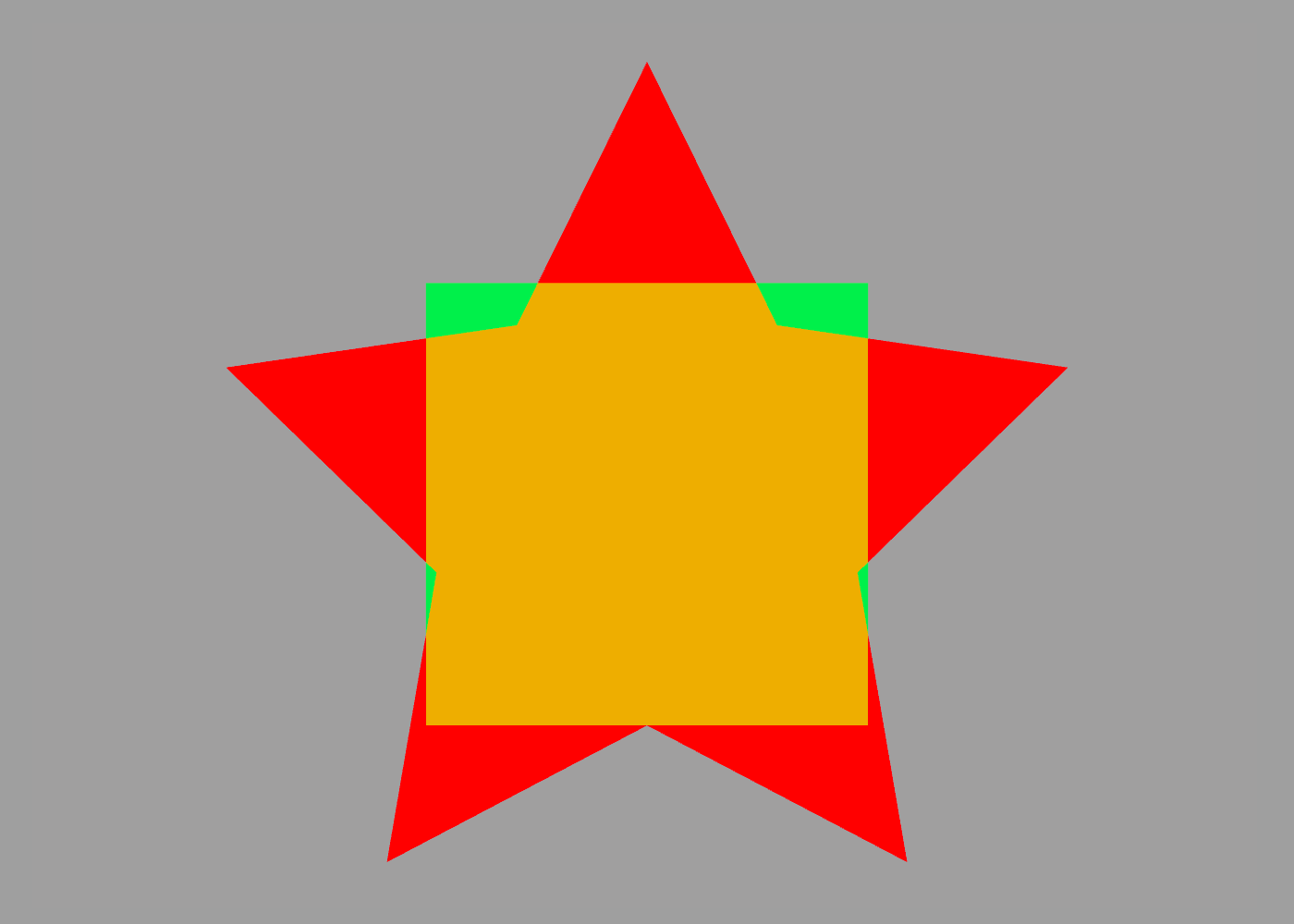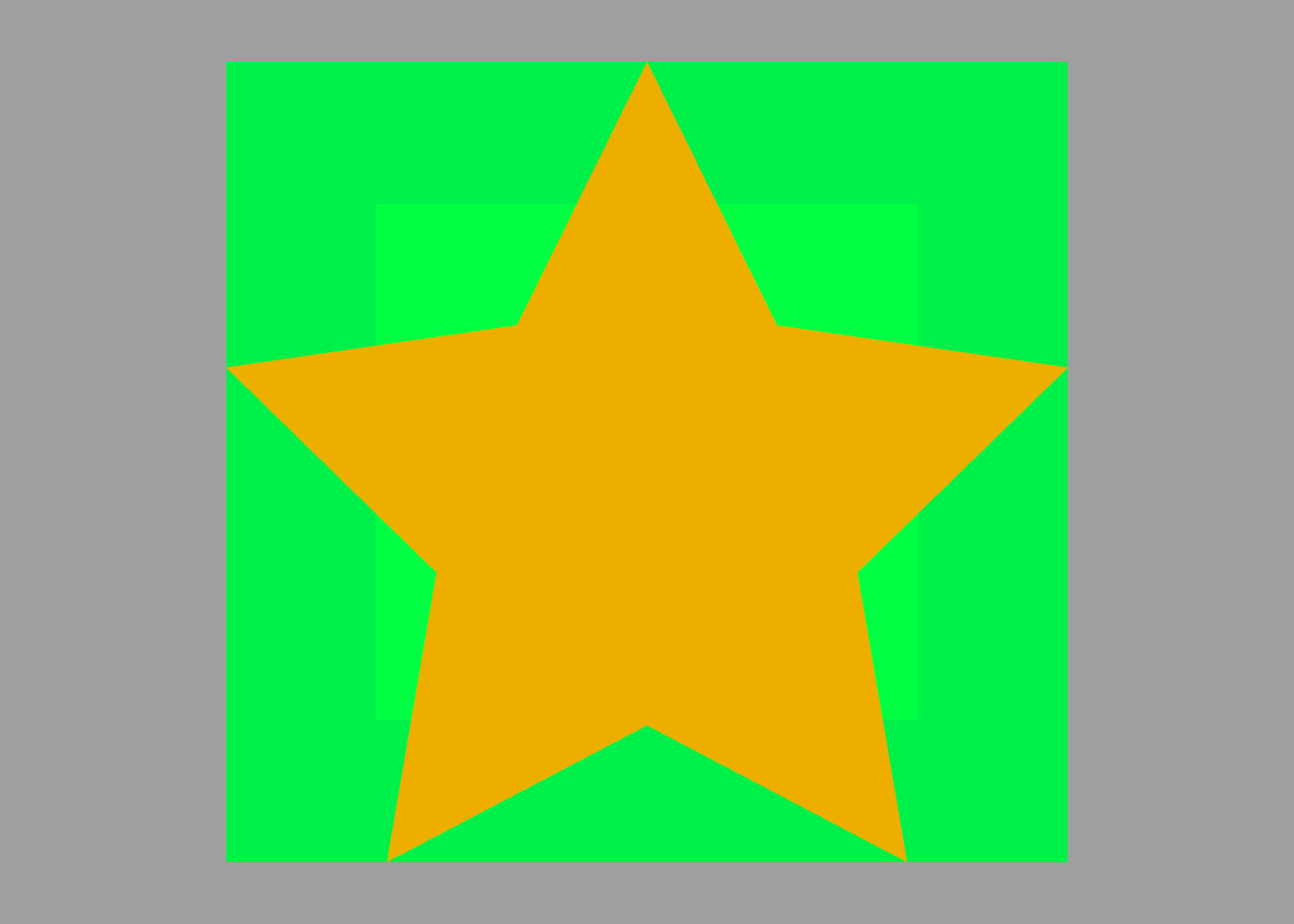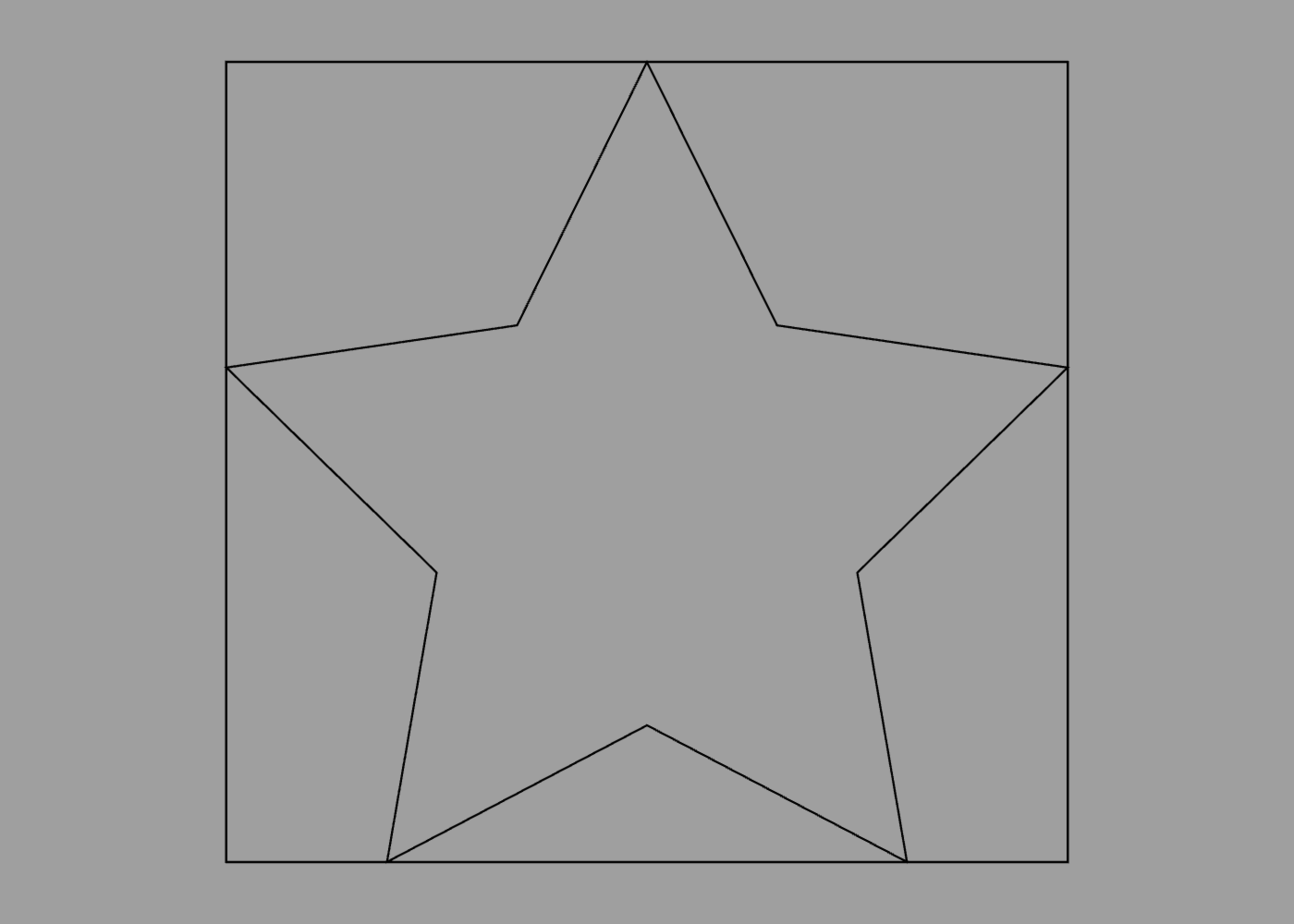# ShapeScript

## Bounds

ShapeScript’s size ands scale commands let you control the relative size of a shape, but sometimes it’s useful to know the exact dimensions.

A cube of size 1 has an easily-predicted size of one world unit square, but what about a more complex shape, such as a 5-pointed star (see the procedural paths and blocks sections for details):

``````define star path {
for 1 to 5 {
point 0 -0.5
rotate 1 / 5
point 0 -1
rotate 1 / 5
}
point 0 -0.5
}

// draw star
extrude {
color red
star
}

// draw cube
cube {
color green 0.5
}
``````We can see that the star is larger than the unit cube, but other than trial-and-error or complex math, how can we get the exact size? This is where the `bounds` member property comes in.

## Mesh Bounds

Paths and meshes both expose a `bounds` property that represents a bounding box around the shape. From this you can get the exact size and position needed to place a box around the star:

``````define star {
...
}

// define star shape
define shape extrude {
color red
star
}

// draw star
shape

// draw box around star
cube {
color green 0.5
position shape.bounds.center
size shape.bounds.size
}
``````## Path Bounds

In the example above we computed the bounds of a solid `mesh` (an extruded star-shaped `path`) but you can also get the bounds of a `path` directly. The following code draws the star path inside its bounding rectangle:

``````define star {
...
}

// draw star
star

// draw rectangle around star
square {
position shape.bounds.center
size shape.bounds.size
}
``````## Bounds Members

The `bounds` member property has the following sub-properties that you can use:

• `min` - The position of the corner of the box with the smallest X, Y and Z values relative to the origin.
• `max` - The position of the corner of the box with the largest X, Y and Z values relative to the origin.
• `center` - The position of the center of the box relative to the origin.
• `size` - The size (width, height and depth) of the box in world units.
• `width` - The width of the box along the X axis (equivalent to `size.width`)
• `height` - The height of the box along the Y axis (equivalent to `size.height`)
• `depth` - The depth of the box along the Z axis (equivalent to `size.depth`)

So, for example, to get the height of a shape, you could use:

``````print someShape.bounds.size.height
``````

or just:

``````print someShape.bounds.height
``````

And to get the X coordinate of its rightmost edge you could use:

``````print someShape.bounds.max.x
``````

Index | Next: Meshes